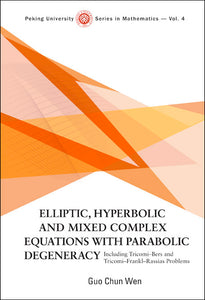# Elliptic, Hyperbolic And Mixed Complex Equations With Parabolic Degeneracy: Including Tricomi-bers And Tricomi-frankl-rassias Problems

Guo Chun Wen

Format: Print Book

ISBN: 9789812779427

• SGD 262.15
Unit price per
Tax included.

In the recent half-century, many mathematicians have investigated various problems on several equations of mixed type and obtained interesting results, with important applications to gas dynamics. However, the Tricomi problem of general mixed type equations of second order with parabolic degeneracy has not been completely solved, particularly the Tricomi and Frankl problems for general Chaplygin equation in multiply connected domains posed by L Bers, and the existence, regularity of solutions of the above problems for mixed equations with non-smooth degenerate curve in several domains posed by J M Rassias.The method revealed in this book is unlike any other, in which the hyperbolic number and hyperbolic complex function in hyperbolic domains, and the complex number and complex function in elliptic domains are used. The corresponding problems for first order complex equations with singular coefficients are first discussed, and then the problems for second order complex equations are considered, where we pose the new partial derivative notations and complex analytic methods such that the forms of the above first order complex equations in hyperbolic and elliptic domains are wholly identical. In the meantime, the estimates of solutions for the above problems are obtained, hence many open problems including the above Tricomi- Bers and Tricomi-Frankl-Rassias problems can be solved.

Format: Hardcover
No of Pages: 456
Imprint: World Scientific
Publication date: 20071214
Series: Peking University Series In Mathematics